N1H111SM's Miniverse

# How Powerful are GNNs?

2020/02/29 ShareMaterials

# Motivation

Despite GNNs revolutionizing graph representation learning, there is limited understanding of their representational properties and limitations. Here, we present a theoretical framework for analyzing the expressive power of GNNs to capture different graph structures. We then develop a simple architecture that is provably the most expressive among the class of GNNs and is as powerful as the Weisfeiler-Lehman graph isomorphism test.

Similar to GNNs, the WL test iteratively updates a given node’s feature vector by aggregating feature vectors of its network neighbors. What makes the WL test so powerful is its injective aggregation update that maps different node neighborhoods to different feature vectors.

Main contributions

• We show that GNNs are at most as powerful as the WL test in distinguishing graph structures.
• We establish conditions on the neighbor aggregation and graph readout functions under which the resulting GNN is as powerful as the WL test.
• We identify graph structures that cannot be distinguished by popular GNN variants, such as GCN and GraphSAGE, and we precisely characterize the kinds of graph structures such GNN-based models can capture.
• We develop a simple neural architecture, Graph Isomorphism Network (GIN), and show that its discriminative/representational power is equal to the power of the WL test.

# Preliminaries

## Weisfeiler-Lehman Test

The graph isomorphism problem asks whether two graphs are topologically identical. This is a challenging problem: no polynomial-time algorithm is known for it yet.

The Weisfeiler-Lehman (WL) test of graph isomorphism is an effective and computationally efficient test that distinguishes a broad class of graphs. Its 1-dimensional form, “naïve vertex refinement”, is analogous to neighbor aggregation in GNNs. The WL test iteratively (1) aggregates the labels of nodes and their neighborhoods, and (2) hashes the aggregated labels into unique new labels. The algorithm decides that two graphs are non-isomorphic if at some iteration the labels of the nodes between the two graphs differ.

# Theoretical Framework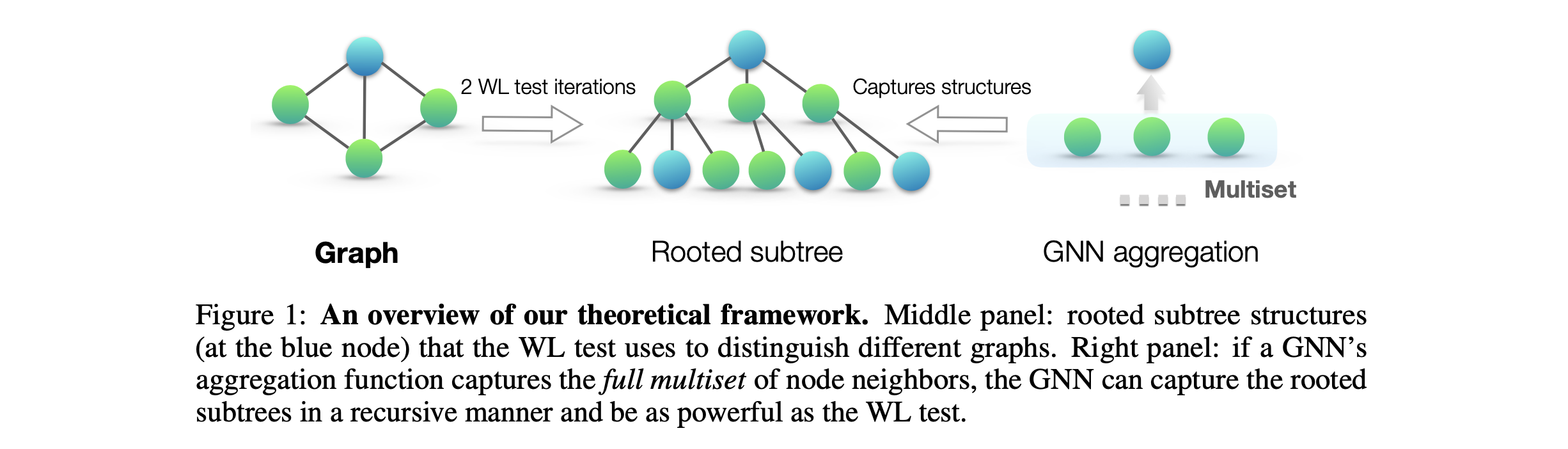A GNN recursively updates each node’s feature vector to capture the network structure and features of other nodes around it, i.e., its rooted subtree structures. For notational simplicity, we can assign each feature vector a unique label in $\{ a, b, c, \cdots \}$. Then, feature vectors of a set of neighboring nodes form a multiset: the same element can appear multiple times since different nodes can have identical feature vectors.

Since subtree structures are defined recursively via node neighborhoods, we can reduce our analysis to the question whether a GNN maps two neighborhoods (i.e., two multisets) to the same embedding or representation. A maximally powerful GNN would never map two different neighborhoods, i.e., multisets of feature vectors, to the same representation. This means its aggregation scheme must be injective.

• 首先定义GNN的aggregation函数是一个作用在由neighborhood定义的multiset上的函数
• 多层的GNN不断地将周围的neighborhood展开变成子树的形式，因为这一递归的定义，我们只需要关注在每一层树的映射上
• 最有鉴别力的GNN的上界就是WL test，这一条件只在aggregation方法是injective时实现，因为这意味着GNN不会将不同的multiset映射到同一个representation上。

# Building Powerful GNN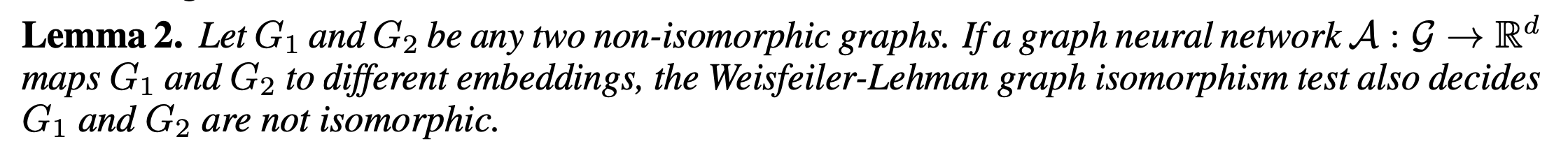A natural follow-up question is whether there exist GNNs that are, in principle, as powerful as the WL test? Our answer, in Theorem 3, is yes: if the neighbor aggregation and graph-level readout functions are injective, then the resulting GNN is as powerful as the WL test.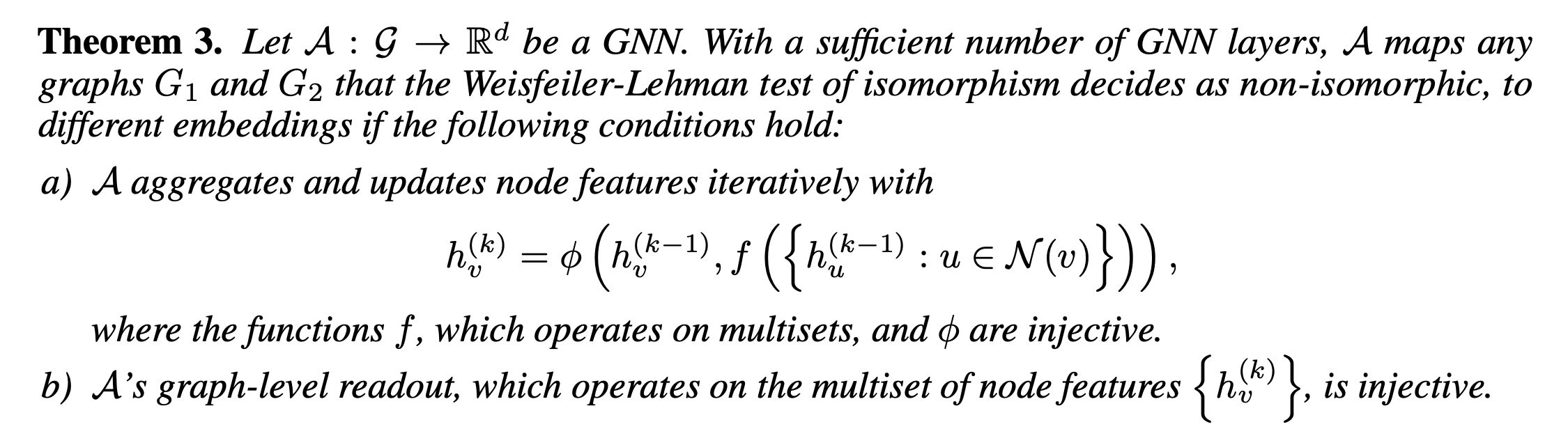For countable sets, injectiveness well characterizes whether a function preserves the distinctness of inputs. Uncountable sets, where node features are continuous, need some further considerations. In addition, it would be interesting to characterize how close together the learned features lie in a function’s image.

## Graph Isomorphism Network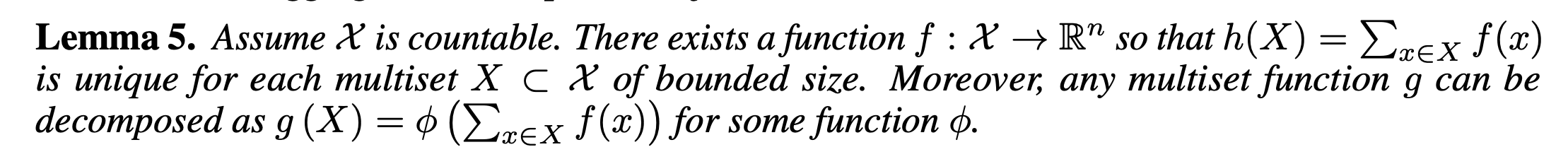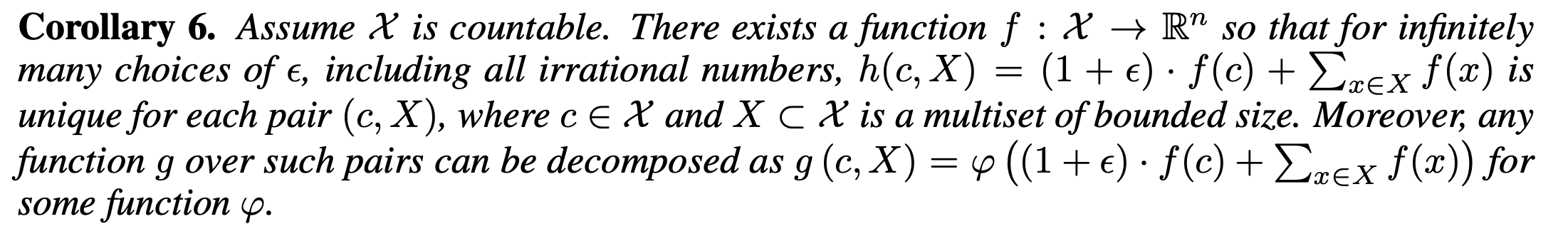## Mean/Max Pooling

What happens if we replace the sum in $h(X)=\sum_{x \in X} f(x)$ with mean or max-pooling as in GCN? Mean and max-pooling aggregators are still well-defined multiset functions because they are permutation invariant. But, they are not injective.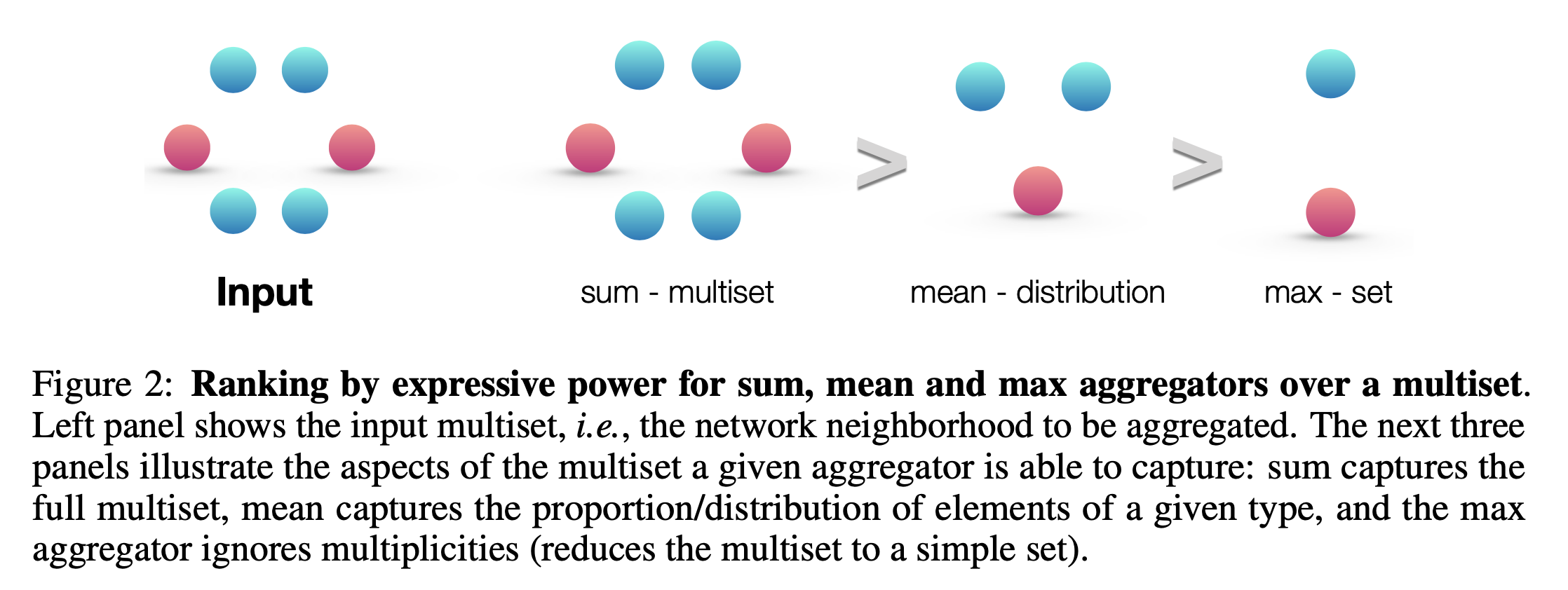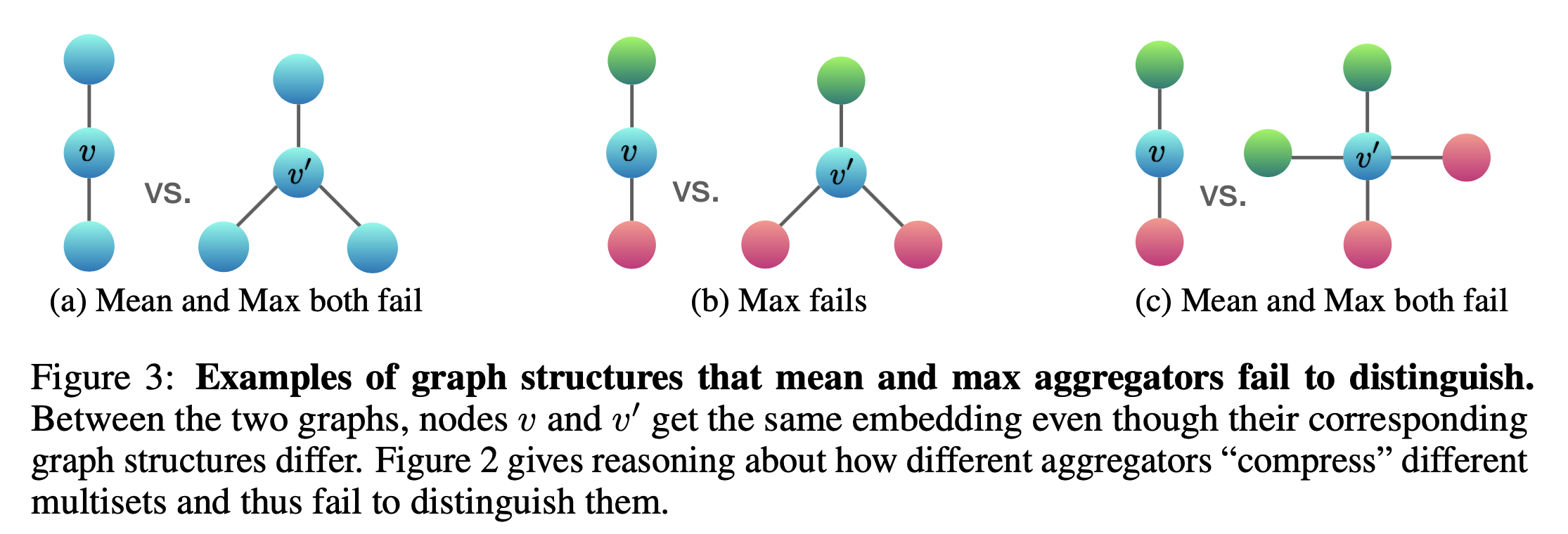### MAX-POOLING LEARNS SETS WITH DISTINCT ELEMENTS

Max-pooling captures neither the exact structure nor the distribution. However, it may be suitable for tasks where it is important to identify representative elements or the “skeleton”, rather than to distinguish the exact structure or distribution. Qi et al. (2017) empirically show that the max-pooling aggregator learns to identify the skeleton of a 3D point cloud and that it is robust to noise and outliers.

# *OpenReview Discussion

### Review1.

My chief concern is equating the Weisfeiler-Lehman test (WL-test) with Weisfeiler-Lehman-type GNNs (WL-GNNs). The WL-test relies on countable set inputs and injective hash functions. Here, the paper is oversimplifying the WL-GNN problem. After the first layer, a WL-GNN is operating on uncountable sets. On uncountable sets, saying that a function is injective does not tells us much about it; we need a measure of how closely packed we find the points in the function’s image (a measure in measure theory, a density in probability). On countable sets, saying a function is injective tells us much about the function. Moreover, the WL-test hash function does not even need to operate over sets with total or even partial orders. As a neural network, the WL-GNN “hash” ( in the paper) must operate over a totally ordered set (\mathbb{R}^n, n > 0). Porting the WL-test argument of “convergence to unique isomorphic fingerprints” to a WL-GNN requires a measure-theoretic analysis of the output of the WL-GNN layers, and careful analysis if the total order of the set does not create attractors when they are applied recursively.

Reply. Validity of equating the WL test operating on countable sets to the WL-GNN operating on uncountable sets. The reviewer makes a great observation that countability of node features is essential and necessary for our theory, and we acknowledge that our current Theorem 3 and Lemma 5 are built on the common assumption that input node features are from a countable universe. We have now made this clear in our paper. We also filled in a technical gap/detail to address R1’s concern that after the first iteration, we are in an uncountable universe: this actually does not happen. We can show that for a fixed aggregation function, hidden node features also form a countable universe, because the countability of input node features recursively propagates into deeper layers. We also added a rigorous proof for this (Lemma 4 in our revised paper). As the reviewer nicely suggests, for the uncountable setting, it would be useful to have measure-theoretic analysis, which we leave for future work. Often input node features in graph classification applications (e.g., chemistry, bioinformatics, social) come from a countable (in fact, finite) universe, so our assumption is realistic. In the revised version, we clearly stated our assumptions at the beginning of Section 3 and have added further discussion on the relation between the WL test and WL-GNN after Theorem 3.

### Review2.

The matrix analysis of the last paragraph also points to another potential problem with the sum aggregator. GIN needs to be shallow. With ReLU activations the reason is simple: for an adjacency matrix $A$, the value of $A^j$ grows very quickly with (diverges). With sigmoid activations, GIN would experience vanishing gradients in graphs with high variance in node degrees.

Reply. Furthermore, the reviewer is concerned with a possibly exploding value due to the sum aggregation, but this can be avoided because we have different learnable neural networks at each layer that can scale down the summed output (also, in practice, we did not observe such explosion).

### Review3.

I understand that GIN provably has more discriminative power than other variants of GNN. But the ability to differentiate non-isomorphic graphs does not necessarily imply better graph classification accuracy, right? Would it be possible to strong discriminative power will backfire for the graph classification? After all, we don’t need to solve graph isomorphism here.

Reply. As we have pointed out in the experiment section, although stronger discriminative power does not directly imply better generalization, it is reasonable to expect that models that can accurately capture graph structures of interest also perform well on test set. In particular, with many existing GNNs, the discriminative power may not be enough to capture graph substructures that are important for classifying graphs. Therefore, we believe strong discriminative power is generally advantageous for graph classification. In our experiments, we empirically demonstrated that our powerful GIN has better generalization as well as better fitting to training datasets compared to other GNN variants. GINs performed the best in general, and achieved state-of-the-art test accuracy. We leave further theoretical investigation of generalization to our future work.